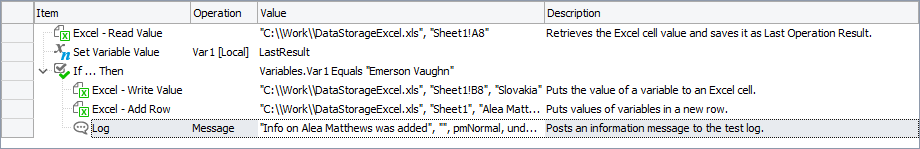# Creating and Opening Excel FilesIn TestComplete tests, you can create Excel files or access already existing ones.

### In Script Tests

The following steps are required:

1. Get access to a file. To create a new file, use the `Excel.Create` method. To access an existing file, use the `Excel.Open` method.

2. Get access to a worksheet. You can specify the worksheet by its title or index. For this purpose, use the `SheetByTitle` or `SheetByIndex` methods respectively.

JavaScript, JScript

function ExcelExample()
{
// Get the data that will be written into an Excel file
var curTime = aqDateTime.Now();
var cpu = Sys.CPUUsage;

var fileName = "C:\\temp\\MyFile.xlsx";

if (aqFile.Exists(fileName))
{
// Open the existing Excel file
var excelFile = Excel.Open(fileName);

if (excelFile.SheetCount > 0)
{
// Get the first sheet of the Excel file
var excelSheet = excelFile.SheetByIndex(0);

// Write the data into a new row of the Excel file
var rowIndex = excelSheet.RowCount + 1;
excelSheet.Cell("A", rowIndex).Value = curTime;
excelSheet.Cell(2, rowIndex).Value = cpu;
}
else
{
Log.Error(aqString.Format("The %s Excel file does not contain any sheets.", fileName));
}
}
else
{
// Create a new Excel file and add a new empty sheet to it
Log.Message(aqString.Format("The %s file does not exist and will be created.", fileName));
excelFile = Excel.Create(fileName);

// Write the data into the first row of the created file
excelSheet.Cell("A", 1).Value = curTime;
excelSheet.Cell(2, 1).Value = cpu;
}

// Save the file to apply the changes
excelFile.Save();
}

Python

``````def ExcelExample():
# Get the data that will be written into an Excel file
curTime = aqDateTime.Now()
cpu = Sys.CPUUsage

fileName = "C:\\temp\\MyFile.xlsx"

if (aqFile.Exists(fileName)):
# Open the existing Excel file
excelFile = Excel.Open(fileName)

if (excelFile.SheetCount > 0):
# Get the first sheet of the Excel file
excelSheet = excelFile.SheetByIndex

# Write the data into a new row of the Excel file
rowIndex = excelSheet.RowCount + 1
excelSheet.Cell["A", rowIndex].Value = curTime
excelSheet.Cell[2, rowIndex].Value = cpu
else:
Log.Error(aqString.Format("The %s Excel file does not contain any sheets.", fileName))
else:
# Create a new Excel file and add a new empty sheet to it
Log.Message(aqString.Format("The %s file does not exist and will be created.", fileName))
excelFile = Excel.Create(fileName)

# Write the data into the first row of the created file
excelSheet.Cell["A", 1].Value = curTime
excelSheet.Cell[2, 1].Value = cpu

# Save the file to apply the changes
excelFile.Save()``````

VBScript

Sub ExcelExample()

' Get the data that will be written into an Excel file
Set curTime = aqDateTime.Now()
cpu = Sys.CPUUsage

fileName = "C:\\temp\\MyFile.xlsx"

If aqFile.Exists(fileName) Then
' Open the existing Excel file
Set excelFile = Excel.Open(fileName)

If excelFile.SheetCount > 0 Then
' Get the first sheet of the Excel file
Set excelSheet = excelFile.SheetByIndex(0)

' Write the data into a new row of the Excel file
rowIndex = excelSheet.RowCount + 1
excelSheet.Cell("A", rowIndex).Value = curTime
excelSheet.Cell(2, rowIndex).Value = cpu
Else
Log.Error(aqString.Format("The %s Excel file does not contain any sheets.", fileName))
End If
Else
' Create a new Excel file and add a new empty sheet to it
Log.Message(aqString.Format("The %s file does not exist and will be created.", fileName))
Set excelFile = Excel.Create(fileName)

' Write the data into the first row of the created file
excelSheet.Cell("A", 1).Value = curTime
excelSheet.Cell(2, 1).Value = cpu
End If

' Save the file to apply the changes
excelFile.Save()
End Sub

DelphiScript

procedure ExcelExample();
var curTime, cpu, fileName, excelFile, excelSheet, rowIndex;
begin
// Get the data that will be written into an Excel file
curTime := aqDateTime.Now();
cpu := Sys.CPUUsage;

fileName := 'C:\\temp\\MyFile.xlsx';

if aqFile.Exists(fileName) then
begin
// Open the existing Excel file
excelFile := Excel.Open(fileName);

if excelFile.SheetCount > 0 then
begin
// Get the first sheet of the Excel file
excelSheet := excelFile.SheetByIndex(0);

// Write the data into a new row of the Excel file
rowIndex := excelSheet.RowCount + 1;
excelSheet.Cell('A', rowIndex).Value := curTime;
excelSheet.Cell(2, rowIndex).Value := cpu;
end
else
Log.Error(aqString.Format('The %s Excel file does not contain any sheets.', fileName));
end
else
begin
// Create a new Excel file and add a new empty sheet to it
Log.Message(aqString.Format('The %s file does not exist and will be created.', fileName));
excelFile := Excel.Create(fileName);

// Write the data into the first row of the created file
excelSheet.Cell('A', 1).Value := curTime;
excelSheet.Cell(2, 1).Value := cpu;
end;

// Save the file to apply the changes
excelFile.Save();
end;

C++Script, C#Script

function ExcelExample()
{
// Get the data that will be written into an Excel file
var curTime = aqDateTime.Now();
var cpu = Sys.CPUUsage;

var fileName = "C:\\temp\\MyFile.xlsx";

if (aqFile["Exists"](fileName))
{
// Open the existing Excel file
var excelFile = Excel["Open"](fileName);

if (excelFile["SheetCount"] > 0)
{
// Get the first sheet of the Excel file
var excelSheet = excelFile["SheetByIndex"](0);

// Write the data into a new row of the Excel file
var rowIndex = excelSheet["RowCount"] + 1;
excelSheet["Cell"]("A", rowIndex)["Value"] = curTime;
excelSheet["Cell"](2, rowIndex)["Value"] = cpu;
}
else
{
Log["Error"](aqString["Format"]("The %s Excel file does not contain any sheets.", fileName));
}
}
else
{
// Create a new Excel file and add a new empty sheet to it
Log["Message"](aqString["Format"]("The %s file does not exist and will be created.", fileName));
excelFile = Excel["Create"](fileName);

// Write the data into the first row of the created file
excelSheet["Cell"]("A", 1)["Value"] = curTime;
excelSheet["Cell"](2, 1)["Value"] = cpu;
}

// Save the file to apply the changes
excelFile["Save"]();
}

### In Keyword Tests

You do not have to add any additional operations to create or open Excel files. TestComplete automatically accesses the file when you start reading data from or writing it to this file.